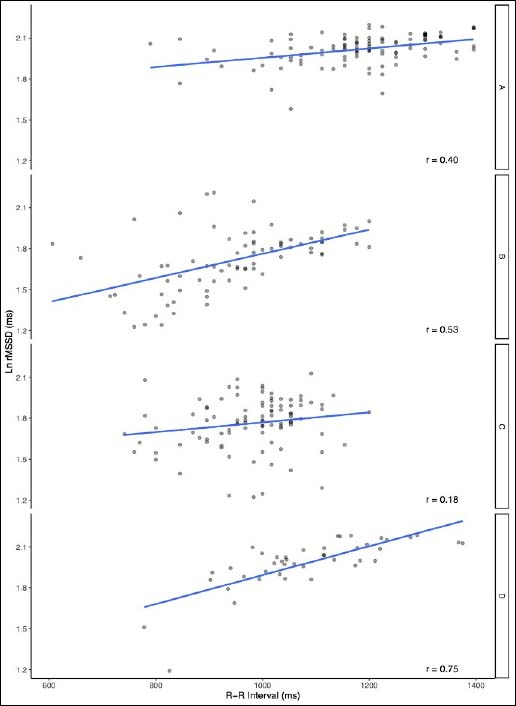Figure 2. The relationship between the natural logarithm of the square of the mean sum of the squared differences between R-R intervals (LnRMSSD) and R-R interval length in four elite international long jump athletes prior and during the 2016 Olympic Games.  LnRMSSD - natural logarithm of the square of the mean sum of the squared differences between R-R intervals, ms – milliseconds.